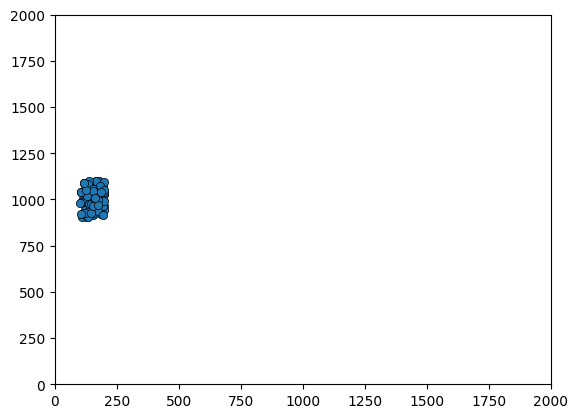Close

COMP0023: Research Software Engineering With Python

Home## The Boids!¶

This section shows an example of using NumPy to encode a model of how a group of birds or other animals moves. It is based on a paper by Craig W. Reynolds. Reynolds calls the simulated creatures "bird-oids" or "boids", so that's what we'll be calling them here too.

### Flocking¶

The aggregate motion of a flock of birds, a herd of land animals, or a school of fish is a beautiful and familiar

part of the natural world... The aggregate motion of the simulated flock is created by a distributed behavioral model much like that at work in a natural flock; the birds choose their own course. Each simulated bird is implemented as an independent actor that navigates according to its local perception of the dynamic environment, the laws of simulated physics that rule its motion, and a set of behaviors programmed into it... The aggregate motion of the simulated flock is the result of the dense interaction of the relatively simple behaviors of the individual simulated birds.

-- Craig W. Reynolds, "Flocks, Herds, and Schools: A Distributed Behavioral Model", Computer Graphics 21 4 1987, pp 25-34

The model includes three main behaviours which, together, give rise to "flocking". In the words of the paper:

• Collision Avoidance: avoid collisions with nearby flockmates
• Velocity Matching: attempt to match velocity with nearby flockmates
• Flock Centering: attempt to stay close to nearby flockmates

### Setting up the Boids¶

Our boids will each have an x velocity and a y velocity, and an x position and a y position.

We'll build this up in NumPy notation, and eventually, have an animated simulation of our flying boids.

In :
import numpy as np


Our positions, for each of our N boids, will be an array, shape $2 \times N$, with the x positions in the first row, and y positions in the second row.

In :
boid_count = 10


We'll want to be able to seed our Boids in a random position.

We'd better define the edges of our simulation area:

In :
limits = np.array([2000, 2000])

In :
positions = np.random.rand(2, boid_count) * limits[:, np.newaxis]
positions

Out:
array([[1322.61236539,  288.73769807, 1681.07537162,  322.70594444,
641.38499356, 1517.25149523,  334.94802661, 1923.99457034,
575.87431142,  592.01104679],
[1905.58214592,  991.21985566, 1596.82817924,  917.50873316,
1759.4808718 ,  627.49083897, 1707.98299175,  599.77153212,
1567.29386623,  375.58638821]])
In :
positions.shape

Out:
(2, 10)

We used broadcasting with np.newaxis to apply our upper limit to each boid. rand gives us a random number between 0 and 1. We multiply by our limits to get a number up to that limit.

In :
limits[:, np.newaxis]

Out:
array([,
])
In :
limits[:, np.newaxis].shape

Out:
(2, 1)
In :
np.random.rand(2, boid_count).shape

Out:
(2, 10)

So we multiply a $2\times1$ array by a $2 \times 10$ array -- and get a $2\times 10$ array.

Let's put that in a function:

In :
def new_flock(count, lower_limits, upper_limits):
width = upper_limits - lower_limits
return (lower_limits[:, np.newaxis] + np.random.rand(2, count) * width[:, np.newaxis])


For example, let's assume that we want our initial positions to vary between 100 and 200 in the x axis, and 900 and 1100 in the y axis. We can generate random positions within these constraints with:

positions = new_flock(boid_count, np.array([100, 900]), np.array([200, 1100]))


But each bird will also need a starting velocity. Let's make these random too:

We can reuse the new_flock function defined above, since we're again essentially just generating random numbers from given limits. This saves us some code, but keep in mind that using a function for something other than what its name indicates can become confusing!

Here, we will let the initial x velocities range over $[0, 10]$ and the y velocities over $[-20, 20]$.

In :
velocities = new_flock(boid_count, np.array([0, -20]), np.array([10, 20]))
velocities

Out:
array([[  9.35059207,   5.5407417 ,   9.09045518,   8.79604464,
9.17006156,   3.70331282,   0.48208397,   0.24639449,
5.59408917,   0.13771209],
[-18.22927385, -17.93604761,   4.05337553,  17.00914858,
14.42829961,  -1.79433551,  -7.08831677,  -2.09658288,
-6.06616577, -10.49551795]])

### Flying in a Straight Line¶

Now we see the real amazingness of NumPy: if we want to move our whole flock according to

$\delta_x = \delta_t \cdot \frac{dv}{dt}$

we just do:

In :
positions += velocities


### Matplotlib Animations¶

So now we can animate our Boids using the matplotlib animation tools. All we have to do is import the relevant libraries:

In :
from matplotlib import animation
from matplotlib import pyplot as plt
%matplotlib inline


Then, we make a static plot, showing our first frame:

In :
# create a simple plot
# initial x position in [100, 200], initial y position in [900, 1100]
# initial x velocity in [0, 10], initial y velocity in [-20, 20]
positions = new_flock(100, np.array([100, 900]), np.array([200, 1100]))
velocities = new_flock(100, np.array([0, -20]), np.array([10, 20]))

figure = plt.figure()
axes = plt.axes(xlim=(0, limits), ylim=(0, limits))
scatter = axes.scatter(positions[0, :], positions[1, :],
marker='o', edgecolor='k', lw=0.5)
scatter

Out:
<matplotlib.collections.PathCollection at 0x7fbe5af2d430>Then, we define a function which updates the figure for each timestep

In :
def update_boids(positions, velocities):
positions += velocities

def animate(frame):
update_boids(positions, velocities)
scatter.set_offsets(positions.transpose())


Call FuncAnimation, and specify how many frames we want:

In :
anim = animation.FuncAnimation(figure, animate,
frames=50, interval=50)


Save out the figure:

In :
positions = new_flock(100, np.array([100, 900]), np.array([200, 1100]))
velocities = new_flock(100, np.array([0, -20]), np.array([10, 20]))
anim.save('boids_1.mp4')


from IPython.display import HTML